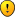## 007 toroidal cross sums sudoku 'jigsaw pieces' - walkthrough

For fans of Killer Sudoku, Samurai Sudoku and other variants

### 007 toroidal cross sums sudoku 'jigsaw pieces' - walkthrough

Another cross sums toroidal. This time with jigsaw pieces as theme.

Fill in the digits 0 (twice) as well as 1 to 7 in every row, column and coloured toroidal region.

Outside the grid you find the sum of the digits you come across first from that side: the number in brackets is the number of digits you have to add up, the other number is the sum.

It has a narrow solving path.Have fun with it.
RichardRichard S

Posts: 24
Joined: 08 June 2006

### walkthrough for this puzzle

As promised, here is my walkthrough for this puzzle
While making the walkthrough, I found out that it's a pretty nasty one! Sorry for that!1.
Realise the sum in every row, column and nonet is 28

2.
R12C3 = {1,2}

3.
R89C3 = {0,4}

4.
R456C3 = {5,6,7}

5.
R7C789 sum = 15 + R7C789 sum = 11 means R168C8 sum = 2 ={0,0,2}
R1C8 = 2
R68C8 = 0

6.
R7C89 = {1,6}

7.
R23C8 = {5,7}

8.
1 in nonet must be R5C2 or R5C4
R5C3 = 5

9.
(4)8 in R5
R5C124 = {0,1,2}

10.
R9C8 = 3

11.
R9C79 = {5,7}

12.
R1C3 = 1
R2C3 = 2

13.
2 in nonet must be in R4C1 or R4C9

14.
2 in nonet must be in RR9C2

15.
(3)10 in C4 must contain a 7 without 2
R123C4 = {0,3,7}

16.
(3)6 in C4 = {0,1,5}
R7C4 = 0
R8C4 = 5
R9C4 = 1

17.
R5C2 = 1
R5C4 = 2
R5C1 = 0

18.
R8C3 = 4
R9C3 = 0
R7C2 = 0

19.
R46C4 = {4,6}

20.
(4)16 in C2 + 1 (R5C2) + 0 (R7C2) + 2 (R9C2) = 19
sum R68C2 = 9 = {3,6}

21.
R3C1 = 6
R1C1 = 3

22.
R3C8 = 7
R2C8 = 5

23.
5 in nonet must be in R46C7
R9C7 = 7
R2C8 = 5

24.
6 in rows 4&6 in columns 3&4
R6C2 = 3
R8C2 = 6

25.
R4C8 cannot be a 6
6 in nonet in R125C9
R7C9 = 1
R7C8 = 6

26.
R5C8 = 4
R4C8 = 1

27.
R3C4 + R3C5 have a sum of 4 inbetween (3)11 and (4)13
R3C4 = 3
R3C5 =1

28.
R3C6 = 0
R3C9 = 4

29.
R29C5 = {4,6}
Because of (4)9 in C5: R9C5 = 4 and R2C5 = 6

30.
R9C1 = 0
R9C6 = 6
R1C9 = 6
R5C7 = 6
R6C1 = 4
R6C4 = 6
R4C4 = 4
R6C3 = 7
R4C3 = 6
R6C7 = 1
R4C7 = 1
R4C7 = 5
R6C7 = 1
R6C9 = 2
R4C1 = 2
R7C5 = 2

31.
(4)9 in C5 = {0,2,3,4}
R6C5 = 0
R8C5 = 3

And the rest are all easy singles...
Richard S

Posts: 24
Joined: 08 June 2006